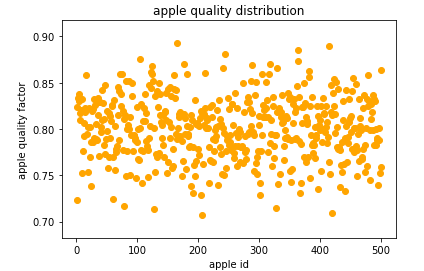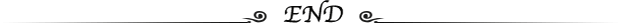# 机器学习储备：概率密度和高斯分布例子解析

01

02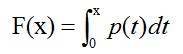F(x)为质量情况X的分布函数，可以看到分布函数是一个区间长度上概率密度的累计。

03

data = np.array([ 0.79,  0.78,  0.8 ,  0.79,  0.77,  0.81,  0.74,  0.85,  0.8 ,

0.77,  0.81,  0.85,  0.85,  0.83,  0.83,  0.8 ,  0.83,  0.71,

0.76,  0.8 ])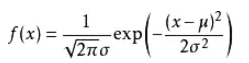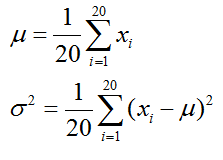import numpy as np

import matplotlib.pyplot as plt

#均值

def average(data):

return np.sum(data)/len(data)

#标准差

def sigma(data,avg):

sigma_squ = np.sum(np.power((data-avg),2))/len(data)

return np.power(sigma_squ,0.5)

#gaussian-distribution prob

def prob(data,avg,sig):

sqrt_2pi = np.power(2*np.pi,0.5)

coef = 1/(sqrt_2pi*sig)

powcoef = -1/(2*np.power(sig,2))

mypow = powcoef * (np.power((data-avg),2))

return coef*(np.exp(mypow))

#样本数据

data = np.array([ 0.79,  0.78,  0.8 ,  0.79,  0.77,  0.81,  0.74,  0.85,  0.8 ,

0.77,  0.81,  0.85,  0.85,  0.83,  0.83,  0.8 ,  0.83,  0.71,

0.76,  0.8 ])

#根据样本求高斯分布的平均数

ave = average(data)

#根据样本求高斯分布的标准差

sig = sigma(data,ave)

#拿到数据

x = np.arange(0.5,1.0,0.01)

p = prob(x,ave,sig)

#绘制函数

plt.plot(x,p)

plt.grid()

plt.xlabel('apple quality factor')

plt.ylabel('prob density')

plt.yticks(np.arange(0,12,1))

plt.title('Gaussian distribution')

plt.show()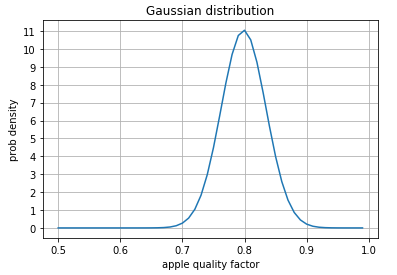1. 可以看到苹果质量在平均数处，大约等于0.8时，取到概率密度的最大值，因为数据样本较少，所以0.5~0.7处概率密度很小。

2. 苹果的质量集中在平均数附近区域

3. 概率密度与x轴所围成的面积为1，等于概率的总和。

4. p值，即概率密度的值不是一定小于1，它和概率的取值不一致，但是概率密度可以理解成概率，也就是说概率是概率密度量纲后的变量，具有相似的意义。

#根据计算出来的均值和方差

data1000 = np.random.normal(0.8,0.036,500)

plt.scatter(np.arange(1,501),data1000,c='orange')

plt.xlabel('apple id')

plt.ylabel('apple quality factor')

plt.title('apple quality distribution')

plt.show()